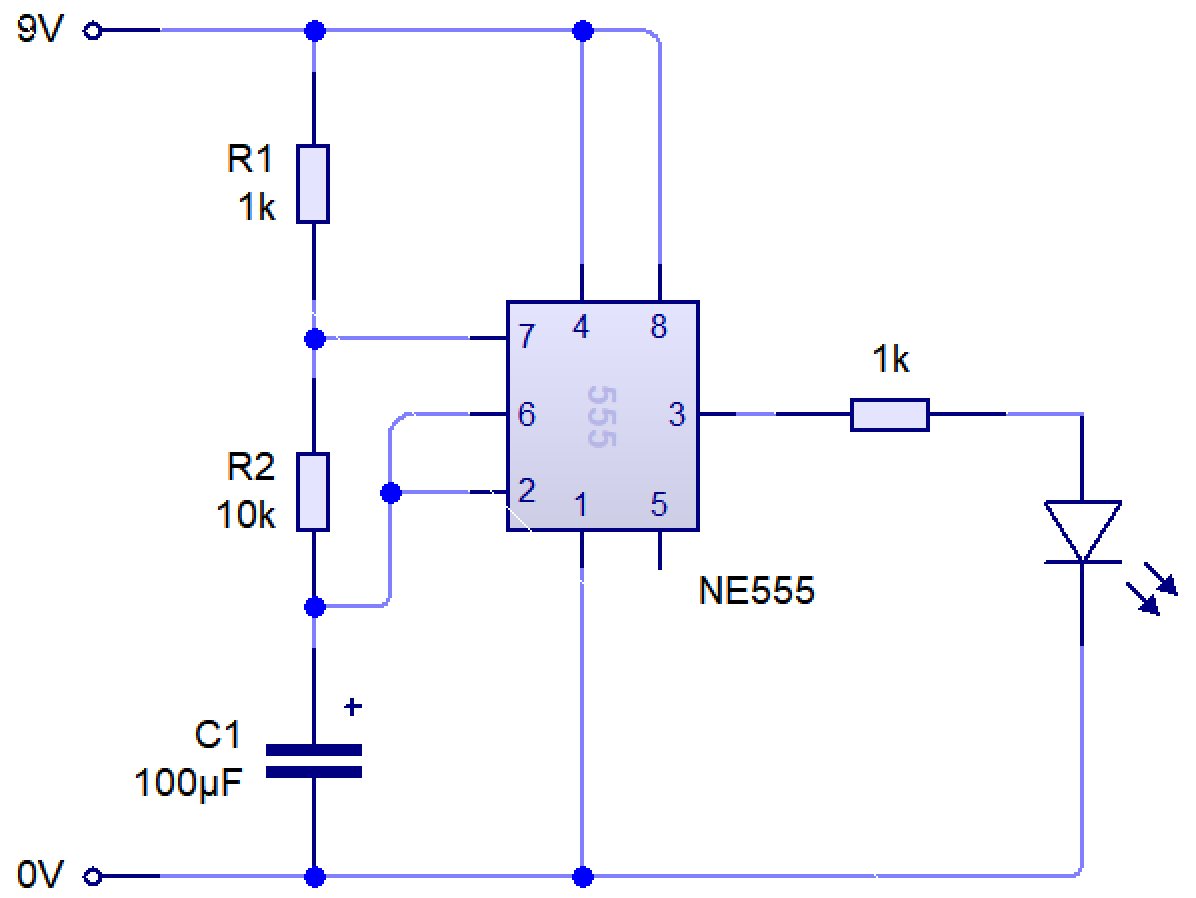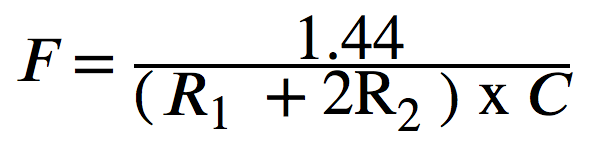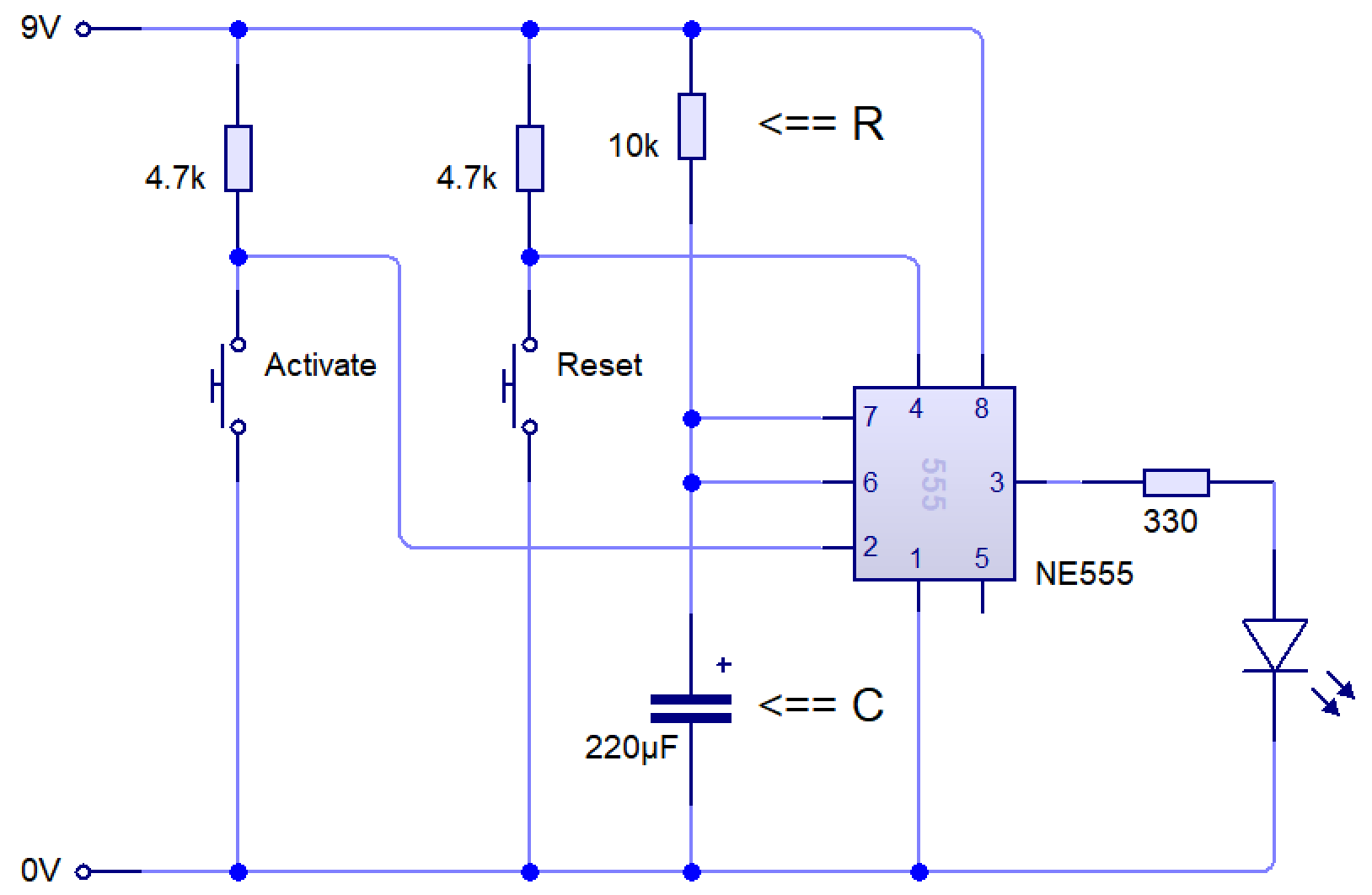# Egg Timer

### Contextual Challenge (the problem that needs solving)

You have decided to make a surprise breakfast for someone for random act of kindness day. As part of that you know they like boiled eggs. You are terrible at time keeping and know that you will probably put the egg on and then forget about it!

The below table helps us to prioritise the requirements for our egg timer, this table is known as a SPECIFICATION.

### Could

Have the ability to start sounding after a certain amount of time Use a potentiometer have a custom designed and made housing
Use light as well as sound to show the alarm Be able to set the time for any value between 5-10 minutes
Be inexpensive i.e. no microcontroller Low power so it lasts a long time.
Loud enough to be heard Have a method of choosing the amount of time

### Learn It

• Boiling an egg requires perfect timing.
• There are many ways to create a timing circuit, but one of the simplest ways is to use a 555 timer circuit.
• The 555 timer circuit relies on a basic electronic timing circuit which uses resistors and capacitors to use time in different ways.
• A capacitor takes time to charge. We can use this, along with a resistor to create different time delays.
• It's output pin (3) can be made to change its behaviour with these two simple components.
• This is the basis of this project.

### Learn It

• A 555 timer can be made to work in a few different ways by changing the circuit slightly.• The circuit diagram above is called `astable`. You can see it running live below.

• The term 'astable' means that the output pin has no stable state; it will constantly flip between being on and off.
• We can calculate how long it stays on (high) and off (low) for by using a few formulae.
• We can calculate how long the output will stay on (high) for in seconds, with:• We can see how long the output will be low for (in seconds) with:• Finally, we can calculate the frequency (number of on/off cycles per second, measured in Hertz) with:• In the example above, resistor 1 has a value of 1k Ohms. 1k is shorthand for 1000, so R1 = 1000
• Resistor 2 has the value 10k, so R2 = 10000
• The capactor (C) has the value 100 μF. μF is shorthand for 'microfarads'.
• You may already know from science that putting a μ before a measurement means that what we are actually writing is that number x10-6.
• So C = 100 μF = 100 x 10-6 = 0.000100 = 0.0001
• We can now calculate the characteristics of our circuit.
• Time High = 0.7 x (1000 + 10000) x 0.0001
• Time High = 0.77s
• Time Low = 0.7 x 10000 x 0.0001
• Time Low = 0.7s
• Frequency = 1.44 / ((1000 + (2x10000) x 0.0001))
• Frequency = 1.44 / ((1000 + 20000) x 0.0001)
• Frequency = 1.44 / (21000 x 0.0001)
• Frequency = 1.44 / 2.1 = 0.69Hz
• These are handy for all sorts of applications where you want something to flip between on and off constantly. E.g.
• A strobe lighting system for a theatre; the light is flipped on and off several times per second.
• A sound generator pulsing a speaker (555 timers can pulse thousands of times a second).
• A daily automatic fish food dispenser (they can also wait up to about three days between going from off to on).
• A speed controller for a model aeroplane (pulsing a motor on and off swiftly will make it run more slowly).
• A set of lights on top of an emergency response vehicle.
• The Year 7 555 piano. Different resistors make the pitch different. It doesn't have to always be an LED.

### Learn It

• Another way is called 'monostable', as the circuit has one stable state: Being turned off.• In this setup, the output pin (3) stays off until an input (e.g. a switch) is triggered.
• Once triggered, the output is turned on for a set time, then goes back to being off again.
• We can calculate the time delay using a simple formula, T = 1.1 x R x C
• In the example above, R is 10k (so R = 10000)
• C is 220μF. Written in Farads, this is 220 x 10-6 = 0.000220.
• T = 1.1 x 10000 x 0.00022
• T = 2.42 seconds.
• These are handy for all sorts of applications where you want something to happen for a set period of time. E.g.
• A patio heater which is turned on for 2 minutes when a switch is pressed.
• A security door for a block of flats, where the door lock is released for 5 seconds when the owner 'buzzes you in'.
• A door bell, where the bell rings for 3 seconds when someone pushes the bell switch.
• A hand drier, where the heater and fan are energised for 15 seconds when someone waves their hand under the sensor.
• An outdoor security light, where a floodlight is energised for a minute when someone walks past.

### Try It

• Try building the `Monostable circuit` above using circuit wizard.
• Change the value of `R` and see how it affects the speed at which the LED flashes.